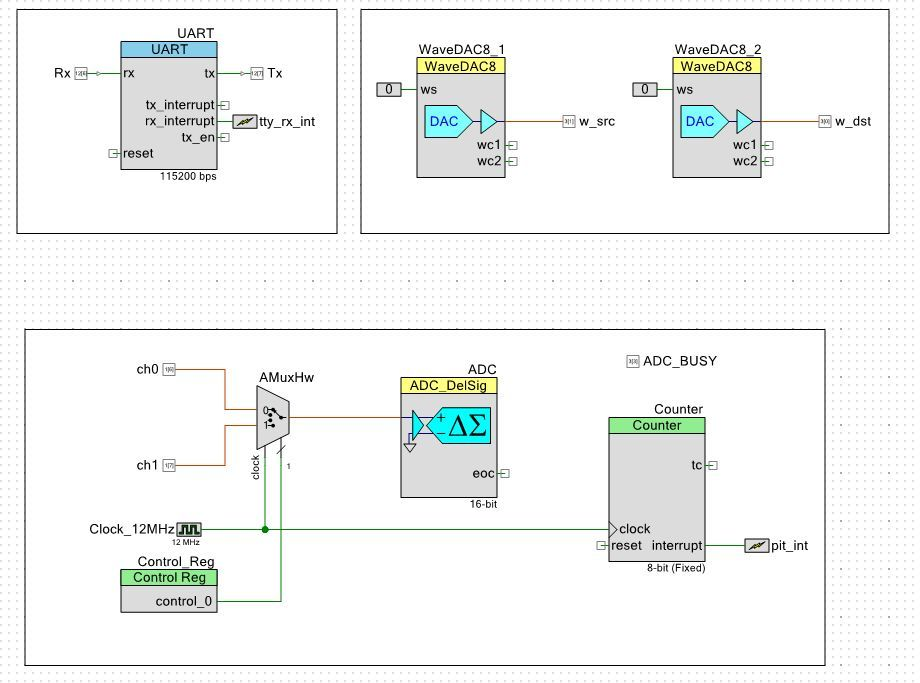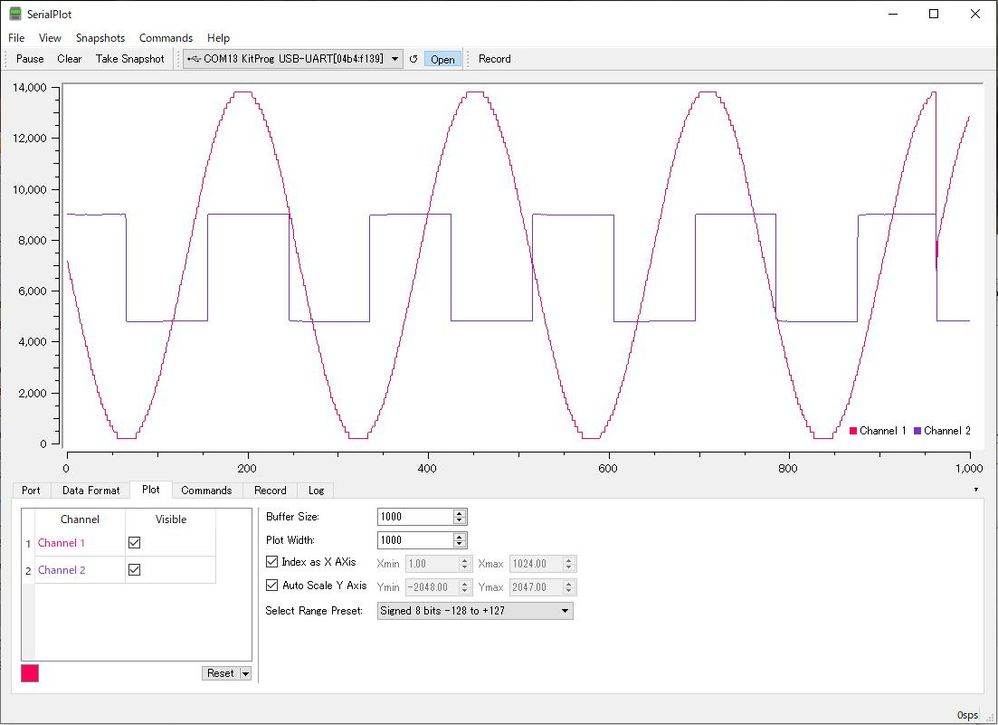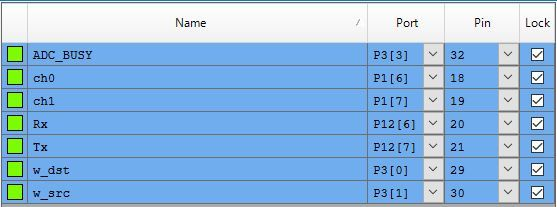## A Sample of Multiplexing input of ADC_DelSig (CY8CKIT-059)Level 9
Level 9# A Sample of Multiplexing input of ADC_DelSig (CY8CKIT-059)

Hi,

We were wondering if we can have multiplexed input for ADC_DelSig.

So I tried one with CY8CKIT-059.

schematic

To test the behavior, I also added a couple of WaveDAC8s

One generates a sine wave, and the other generates a square wave.

And AMuxHw is controlled from Control_Reg.

To test, please connect following pins with jumper wires

P3 and P1

P3 and P1The result viewed via SerialPlot wasSo, yes, it is working.

Pinsmain.c

Note: As usual, I cheated by using my tty_utils library,

But you could replace

tty_init() with UART_Start()

print() with UART_PutString()

if you don't want to use that library.

(You may need to comment out splash() though)

====================

#include "project.h"

#include "stdio.h"

#include "tty_utils.h"

#define DATA_NUM 1024

uint16_t data[DATA_NUM] ;

volatile int pit_flag = 0 ;

volatile int ch = 0 ;

volatile int data_index = 0 ;

volatile int data_full = 0 ;

CY_ISR(pit_isr)

{

pit_flag = 1 ;

Control_Reg_Write(ch) ;

ch++ ;

if (ch > 1) {

data_index++ ;

ch = 0 ;

if (data_index >= DATA_NUM) {

Counter_Stop() ;

data_full = 1 ;

data_index = 0 ;

}

}

}

void init_hardware(void)

{

CyGlobalIntEnable; /* Enable global interrupts. */

tty_init() ;

WaveDAC8_1_Start() ;

WaveDAC8_2_Start() ;

pit_int_ClearPending() ;

pit_int_StartEx(pit_isr) ;

}

int32_t measure(int ch)

{

int32_t value ;

Control_Reg_Write(ch) ;

if (value < 0) {

value = 0 ;

}

return(value) ;

}

int main(void)

{

int i ;

init_hardware() ;

splash("DelSig ADC 2ch mux test") ;

Counter_Start() ;

for(;;) {

if (data_full) {

data_full = 0 ;

for (i = 0 ; i < DATA_NUM ; i++ ) {

snprintf(str, STR_BUF_LEN, "%d %d\n\r", data, data) ;

print(str) ;

CyDelay(1) ;

}

Counter_Start() ;

}

}

}

====================

moto

0 Replies